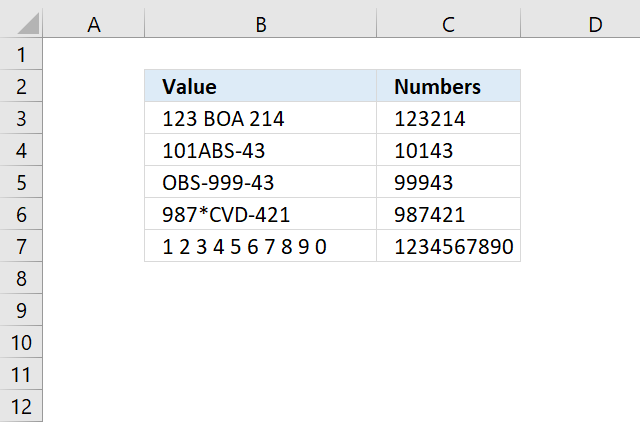Author: Oscar Cronquist Article last updated on December 16, 2021The following array formula, demonstrated in cell C3, extracts all numbers from a cell value:

=TEXTJOIN(, 1, TEXT(MID(B3, ROW(\$A\$1:INDEX(\$A\$1:\$A\$1000, LEN(B3))), 1), "#;-#;0;"))

To enter an array formula, type the formula in a cell then press and hold CTRL + SHIFT simultaneously, now press Enter once. Release all keys.

The formula bar now shows the formula with a beginning and ending curly bracket telling you that you entered the formula successfully. Don't enter the curly brackets yourself.

If your cell contains more than a 1000 characters change this part of the formula \$A\$1:\$A\$1000 to perhaps \$A\$1:\$A\$2000 depending on how many characters you have.

### Explaining array formula in cell C3

#### Step 1 - Count characters

The LEN function returns the number of characters in cell C3, so we can split each character into an array.

LEN(B3)

becomes

LEN("123 BOA 214")

returns 12.

#### Step 2 - Build cell reference

The INDEX function allows us to build a cell reference with as many rows as there are characters in cell B3.

\$A\$1:INDEX(\$A\$1:\$A\$1000, LEN(B3))

becomes

\$A\$1:INDEX(\$A\$1:\$A\$1000,12)

and returns \$A\$1:\$A\$12.

#### Step 3 - Create numbers based on row number of each cell in cell reference

The ROW function converts the cell reference to an array of numbers corresponding to the of each cell.

ROW(\$A\$1:INDEX(\$A\$1:\$A\$1000, LEN(B3)))

becomes

ROW(\$A\$1:\$A\$12)

and returns {1; 2; 3; ... 12}.

#### Step 4 - Create an array

The MID function splits each character in cell B3 into an array.

MID(B3, ROW(\$A\$1:INDEX(\$A\$1:\$A\$1000, LEN(B3))), 1)

becomes

MID(B3, {1; 2; 3; ... 12}, 1)

and returns {"1";"2";"3"; ... ;" "}

#### Step 5 - Filter out text letters

The TEXT function returns numerical values but leaves all other characters blank if you use the following pattern in the format_text argument: "#;-#;0;"

TEXT(valueformat_text)

TEXT(MID(B3, ROW(\$A\$1:INDEX(\$A\$1:\$A\$1000, LEN(B3))), 1), "#;-#;0;")

becomes

TEXT({"1";"2";"3";" ";"B";"O";"A";" ";"2";"1";"4";" "}, "#;-#;0;")

and returns

{"1"; "2"; "3"; ""; ""; ""; ""; ""; "2"; "1"; "4"; ""}.

#### Step 6 - Join numbers

The TEXTJOIN function introduced in Excel 2016 allows you to easily concatenate an array for values. In this case, it also ignores blank values in the array.

TEXTJOIN(, 1, TEXT(MID(B3, ROW(\$A\$1:INDEX(\$A\$1:\$A\$1000, LEN(B3))), 1), "#;-#;0;"))

becomes

TEXTJOIN(, 1, {"1"; "2"; "3"; ""; ""; ""; ""; ""; "2"; "1"; "4"; ""})

and returns 123214 in cell C3.

### Get Excel *.xlsx file

How to extract numbers from a cell value.xlsx